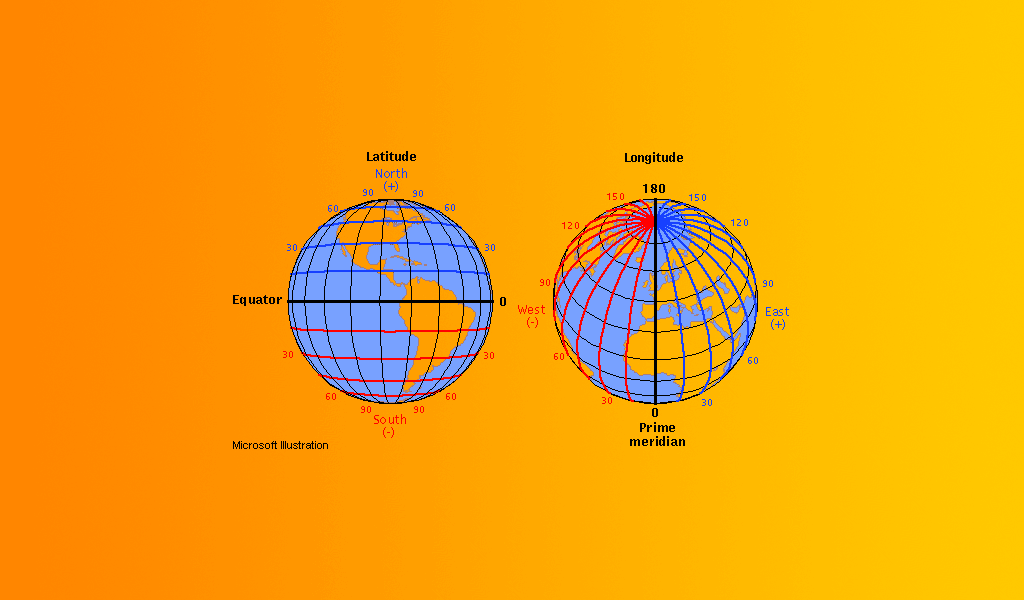# Fastest way to calculate distance between coordinatesI’m always facing the problem of calculating the distance between two points on the surface of the Earth. Most of my readers will already be aware of the Haversine formula, so let’s dive in and see how many earth shattering speed enhancements we can make.

## Good old haversine

Haversine is a pretty solid way to calculate distances. Although it assumes the earth is a sphere, it sacrifices very little and reaches a good level of speed. Despite it being the more performant among the top distance algorithms, the haversine still involves 7 computationally expensive trigonometric functions (4 sines, 2 cosines, 1 arc tangent, 2 powers of two, and 2 square roots) and looks like this:

``````const R = 6378.137;
const toRad = n => n * Math.PI / 180;

export const getDistance = (a, b) => {
const dLat = toRad(b.lat - a.lat);
const dLon = toRad(b.lng - a.lng);
const f = Math.pow(Math.sin(dLat / 2), 2) + (Math.pow(Math.sin(dLon / 2), 2) * Math.cos(aLat) * Math.cos(bLat));
const c = 2 * Math.atan2(Math.sqrt(f), Math.sqrt(1 - f));
return R * c;
};
``````

I benchmarked the haversine taking 1.413s to process 5 million coordinate pairs.

## Optimizing for small distances: the fast haversine

My own use case allowed me to take some additional shortcuts. I got rid of the Sin calculations. On top of that, the Cos of two close latitudes is approximately the Cos of its average. That allowed me to remove one Cos and assume some error. Finally, the two Pow were inlined, so no need to call an external generic power function if only to multiply a number by itself. The final algorithm, with some additional inlining to optimize even more, looks like this:

``````const R = 6378.137;
const PI_360 = Math.PI / 360;

export const getDistance = (a, b) => {
const cLat = Math.cos((a.lat + b.lat) * PI_360);
const dLat = (b.lat - a.lat) * PI_360;
const dLon = (b.lng - a.lng) * PI_360;
const f = dLat * dLat + cLat * cLat * dLon * dLon;
const c = 2 * Math.atan2(Math.sqrt(f), Math.sqrt(1 - f));
return R * c;
};
``````

Benchmarking 5 million coordinate pairs took 303.719ms. We are doing well with impressive gains. This one took only 21% of the time compared to baseline.

## Reaching the end-game, the sloppy haversine

After finding the fast haversine formula, I could not stand still. I knew there was one more way to push the formula even further by revisiting our good friend trigonometry. By now we know that haversine exists to accommodate the earth’s spherical math. However when we are looking close to the earth’s surface it no longer appears as a sphere and instead looks flat. So if we can find a factor to multiply by the longitude delta, and a factor to multiply by the latitude delta, we can use trigonometry as if we are dealing with a flat plane. This is what is known as a projection which is when you flatten the latitude and longitude into x and y coordinates that represent kilometers. That code is this magnificent sloppy boy:

``````const kRad = Math.PI / 180;
export const getDistance = (a, b) => {
const kx = Math.cos(a.lat * kRad) * 111.321;
const dx = (a.lng - b.lng) * kx;
const dy = (a.lat - b.lat) * 111.139;
return Math.sqrt((dx * dx) + (dy * dy));
};
``````

This new algorithm takes a mere square root and cosine call and completes 5 million coordinate pairs in a break neck speed of 215.79ms which is only 15% of the baseline!

## Going even further for a specific use case

Usually we want to calculate the distance of your current location to many other locations, so your current location will be the same for each calculation. In the sloppy haversine, the variable `kx`, which is the factor to multiply the `x` coordinate by, will not change unless the first location changes. We can make a closure that reuses the cosine, so that each individual comparison uses only one square root call:

``````const kRad = Math.PI / 180;
export const ruler = (a) => {
const kx = Math.cos(a.lat * kRad) * 111.321;
return (b) => {
const dx = (a.lng - b.lng) * kx;
const dy = (a.lat - b.lat) * 111.139;
return Math.sqrt((dx * dx) + (dy * dy));
}
};
``````

## Removing the square root

Hilariously, if you are only comparing distances and not displaying them, there is no need for the square root. Simply comparing the result of `(dx * dx) + (dy * dy)` will do. And with the last math call gone, we can plainly see here that with just a little work, we can avoid doing any work, which is kind of my life philosophy.

So, we can conclude that haversine is very generalized, and you should choose algorithms knowing how they work, not just blindly importing them!